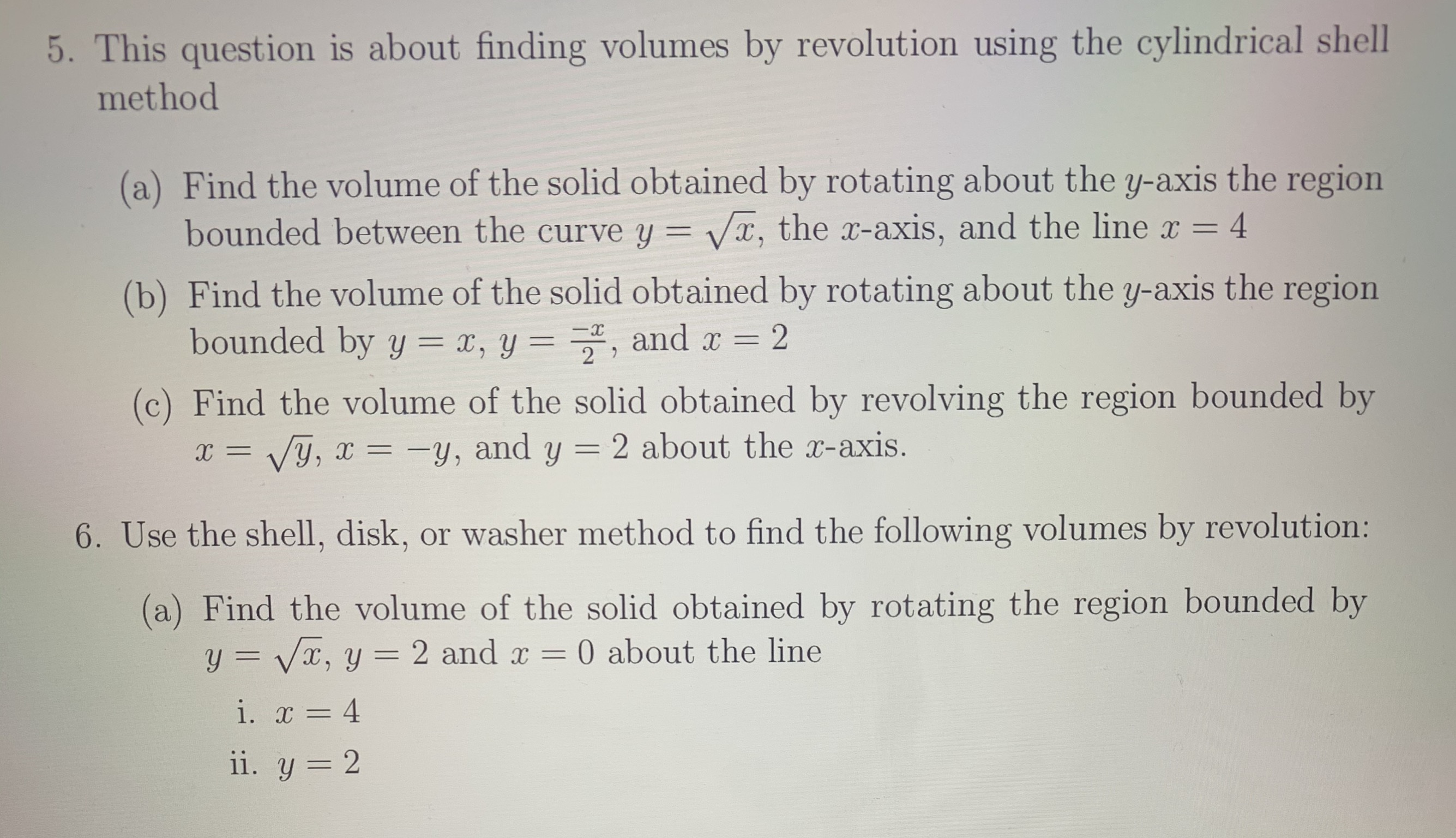# 5. This question is about finding volumes by revolution using the cylindrical shellmethod(a) Find the volume of the solid obtained by rotating about the y-axis the regionbounded between the curve y = x, the x-axis, and the line x4(b) Find the volume of the solid obtained by rotating about the y-axis the regionbounded by y = x, y = , andx 22(c) Find the volume of the solid obtained by revolving the region bounded byy, and y = 2 about the x-axis.Vy,6. Use the shell, disk, or washer method to find the following volumes by revolution:(a) Find the volume of the solid obtained by rotating the region bounded byy=Vx, y0 about the line2 and xi. 4ii. y 2

Question
12 views

Find the Volume of 5 (B) using the shell method.help_outlineImage Transcriptionclose5. This question is about finding volumes by revolution using the cylindrical shell method (a) Find the volume of the solid obtained by rotating about the y-axis the region bounded between the curve y = x, the x-axis, and the line x 4 (b) Find the volume of the solid obtained by rotating about the y-axis the region bounded by y = x, y = , and x 2 2 (c) Find the volume of the solid obtained by revolving the region bounded by y, and y = 2 about the x-axis. Vy, 6. Use the shell, disk, or washer method to find the following volumes by revolution: (a) Find the volume of the solid obtained by rotating the region bounded by y=Vx, y 0 about the line 2 and x i. 4 ii. y 2 fullscreen
check_circle

Step 1

Let R be the region bounded by the curve y = x, y = –x/2 and x = 2.

This implies that y varies from&ndas...

### Want to see the full answer?

See Solution

#### Want to see this answer and more?

Solutions are written by subject experts who are available 24/7. Questions are typically answered within 1 hour.*

See Solution
*Response times may vary by subject and question.
Tagged in

### Calculus